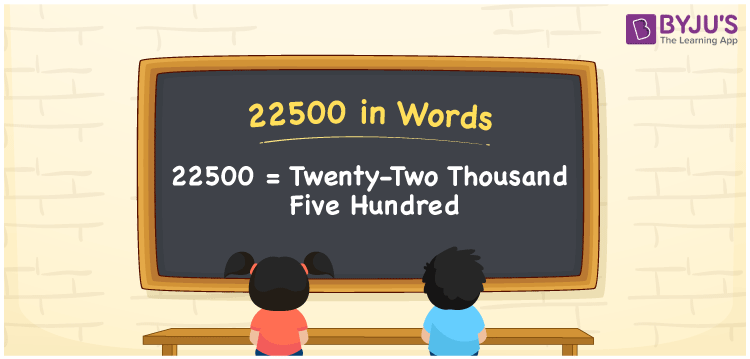# 22500 in Words

22500 in words is written as “Twenty-two thousand five hundred”. For example, the cheque of Rs. 22500 is given by Rupees Twenty-two thousand five hundred only. In Mathematics, 22500 is also a cardinal number, that is to represent the quantity or value of something.

 22500 in Words Twenty-two thousand five hundred Twenty-two thousand five hundred in Numbers 22500

## 22500 in English Words## How to Write 22500 in Words?

22500 is a five-digit number that can be written in words, by knowing the position of each digit in the number. This can be done using a place value chart.

 Ten thousands Thousands Hundreds Tens Ones 2 2 5 0 0

We can see, from the above table, the positions of digits are:

• 2 → Ten thousands
• 2 → Thousands
• 5 → Hundreds
• 0 → Tens
• 0 → Ones

Hence, when we read the number from right to left, it is given by Twenty-two thousand five hundred. Learn more about numbers in words at BYJU’S.

### Expanded Form of 22500

We can write the expanded form as:

2 × Ten thousand + 2 × Thousand + 5 × Hundred + 0 × Ten + 0 × One

= 2 × 10000 + 2 × 1000 + 5 × 100 + 0 × 10 + 0 × 1

= 20000 + 2000 + 500 + 0 + 0

= 22500

= Twenty-two thousand five hundred

22500 is the natural number that is succeeded by 22499 and preceded by 22501. Learn more about the number 22500 below:

• 22500 in Words – Twenty-two thousand five hundred
• Is 22500 an odd number? – No
• Is 22500 an even number? – Yes
• Is 22500 a perfect square number? – Yes (√22500 = 150)
• Is 22500 a perfect cube number? – No
• Is 22500 a prime number? – No
• Is 22500 a composite number? – Yes

## Frequently Asked Questions on 22500 in words

Q1

### What is 22500 in words?

22500 in words is given by Twenty-two thousand five hundred.
Q2

### What is the place value of 5 in 22500?

The place value of 5 in 22500 is hundred.
Q3

### How to write 22500 in English words?

22500 in English is expressed as Twenty-two thousand five hundred